# evaluateHeatFlux

Evaluate heat flux of a thermal solution at nodal or arbitrary spatial locations

## Syntax

``````[qx,qy] = evaluateHeatFlux(thermalresults,xq,yq)``````
``````[qx,qy,qz] = evaluateHeatFlux(thermalresults,xq,yq,zq)``````
``[___] = evaluateHeatFlux(thermalresults,querypoints)``
``[___] = evaluateHeatFlux(___,iT)``
``````[qx,qy] = evaluateHeatFlux(thermalresults)``````
``````[qx,qy,qz] = evaluateHeatFlux(thermalresults)``````

## Description

example

``````[qx,qy] = evaluateHeatFlux(thermalresults,xq,yq)``` returns the heat flux for a thermal problem at the 2-D points specified in `xq` and `yq`. This syntax is valid for both the steady-state and transient thermal models.```

example

``````[qx,qy,qz] = evaluateHeatFlux(thermalresults,xq,yq,zq)``` returns the heat flux for a thermal problem at the 3-D points specified in `xq`, `yq`, and `zq`. This syntax is valid for both the steady-state and transient thermal models.```

example

````[___] = evaluateHeatFlux(thermalresults,querypoints)` returns the heat flux for a thermal problem at the 2-D or 3-D points specified in `querypoints`. This syntax is valid for both the steady-state and transient thermal models.```

example

````[___] = evaluateHeatFlux(___,iT)` returns the heat flux for a thermal problem at the times specified in `iT`. You can specify `iT` after the input arguments in any of the previous syntaxes.The first dimension of `qx`, `qy`, and, in the 3-D case, `qz` corresponds to query points. The second dimension corresponds to time steps `iT`.```

example

``````[qx,qy] = evaluateHeatFlux(thermalresults)``` returns the heat flux for a 2-D problem at the nodal points of the triangular mesh. The first dimension of `qx` and `qy` represents the node indices. The second dimension represents time steps.```

example

``````[qx,qy,qz] = evaluateHeatFlux(thermalresults)``` returns the heat flux for a 3-D thermal problem at the nodal points of the tetrahedral mesh. The first dimension of `qx`, `qy`, and `qz` represents the node indices. The second dimension represents time steps.```

## Examples

collapse all

For a 2-D steady-state thermal model, evaluate heat flux at the nodal locations and at the points specified by `x` and `y` coordinates.

Create a thermal model for steady-state analysis.

`thermalmodel = createpde('thermal');`

Create the geometry and include it in the model.

```R1 = [3,4,-1,1,1,-1,1,1,-1,-1]'; g = decsg(R1,'R1',('R1')'); geometryFromEdges(thermalmodel,g); pdegplot(thermalmodel,'EdgeLabels','on') xlim([-1.5 1.5]) axis equal```Assuming that this geometry represents an iron plate, the thermal conductivity is $79.5\phantom{\rule{0.16666666666666666em}{0ex}}W/\left(mK\right)$.

`thermalProperties(thermalmodel,'ThermalConductivity',79.5,'Face',1);`

Apply a constant temperature of 500 K to the bottom of the plate (edge 3). Also, assume that the top of the plate (edge 1) is insulated, and apply convection on the two sides of the plate (edges 2 and 4).

```thermalBC(thermalmodel,'Edge',3,'Temperature',500); thermalBC(thermalmodel,'Edge',1,'HeatFlux',0); thermalBC(thermalmodel,'Edge',[2 4], ... 'ConvectionCoefficient',25, ... 'AmbientTemperature',50);```

Mesh the geometry and solve the problem.

```generateMesh(thermalmodel); results = solve(thermalmodel)```
```results = SteadyStateThermalResults with properties: Temperature: [1541x1 double] XGradients: [1541x1 double] YGradients: [1541x1 double] ZGradients: [] Mesh: [1x1 FEMesh] ```

Evaluate heat flux at the nodal locations.

```[qx,qy] = evaluateHeatFlux(results); figure pdeplot(thermalmodel,'FlowData',[qx qy])```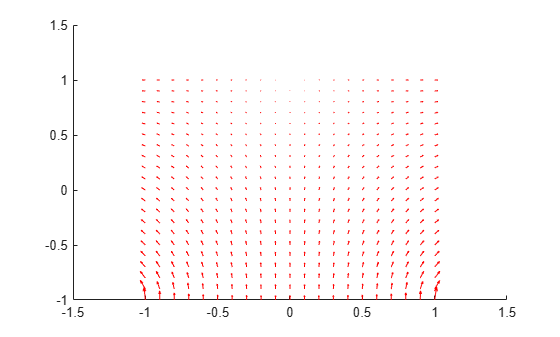Create a grid specified by `x` and `y` coordinates, and evaluate heat flux to the grid.

```v = linspace(-0.5,0.5,11); [X,Y] = meshgrid(v); [qx,qy] = evaluateHeatFlux(results,X,Y);```

Reshape the `qTx` and `qTy` vectors, and plot the resulting heat flux.

```qx = reshape(qx,size(X)); qy = reshape(qy,size(Y)); figure quiver(X,Y,qx,qy)```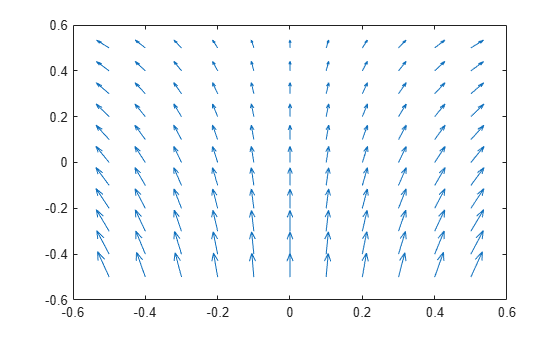Alternatively, you can specify the grid by using a matrix of query points.

```querypoints = [X(:) Y(:)]'; [qx,qy] = evaluateHeatFlux(results,querypoints); qx = reshape(qx,size(X)); qy = reshape(qy,size(Y)); figure quiver(X,Y,qx,qy)```For a 3-D steady-state thermal model, evaluate heat flux at the nodal locations and at the points specified by `x`, `y`, and `z` coordinates.

Create a thermal model for steady-state analysis.

`thermalmodel = createpde('thermal');`

Create the following 3-D geometry and include it in the model.

```importGeometry(thermalmodel,'Block.stl'); pdegplot(thermalmodel,'FaceLabels','on','FaceAlpha',0.5) title('Copper block, cm') axis equal```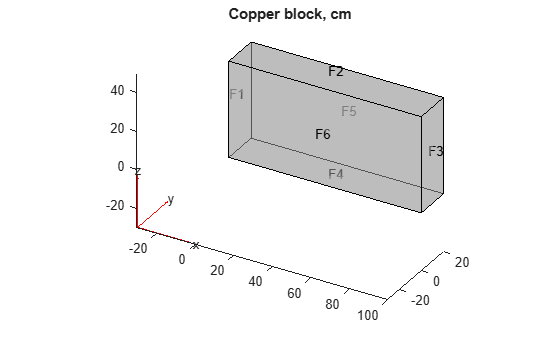Assuming that this is a copper block, the thermal conductivity of the block is approximately $4\phantom{\rule{0.16666666666666666em}{0ex}}W/\left(cmK\right)$.

`thermalProperties(thermalmodel,'ThermalConductivity',4);`

Apply a constant temperature of 373 K to the left side of the block (face 1) and a constant temperature of 573 K to the right side of the block (face 3).

```thermalBC(thermalmodel,'Face',1,'Temperature',373); thermalBC(thermalmodel,'Face',3,'Temperature',573);```

Apply a heat flux boundary condition to the bottom of the block.

`thermalBC(thermalmodel,'Face',4,'HeatFlux',-20);`

Mesh the geometry and solve the problem.

```generateMesh(thermalmodel); thermalresults = solve(thermalmodel)```
```thermalresults = SteadyStateThermalResults with properties: Temperature: [12691x1 double] XGradients: [12691x1 double] YGradients: [12691x1 double] ZGradients: [12691x1 double] Mesh: [1x1 FEMesh] ```

Evaluate heat flux at the nodal locations.

```[qx,qy,qz] = evaluateHeatFlux(thermalresults); figure pdeplot3D(thermalmodel,'FlowData',[qx qy qz])```Create a grid specified by `x`, `y`, and `z` coordinates, and evaluate heat flux to the grid.

```[X,Y,Z] = meshgrid(1:26:100,1:6:20,1:11:50); [qx,qy,qz] = evaluateHeatFlux(thermalresults,X,Y,Z);```

Reshape the `qx`, `qy`, and `qz` vectors, and plot the resulting heat flux.

```qx = reshape(qx,size(X)); qy = reshape(qy,size(Y)); qz = reshape(qz,size(Z)); figure quiver3(X,Y,Z,qx,qy,qz)```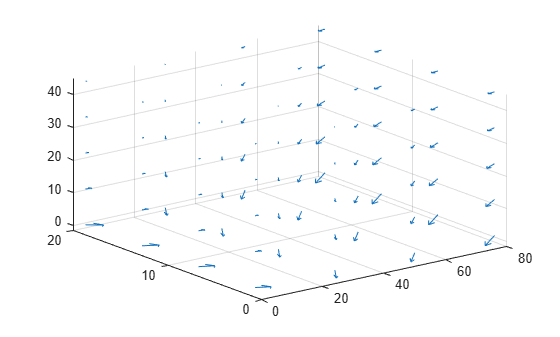Alternatively, you can specify the grid by using a matrix of query points.

```querypoints = [X(:) Y(:) Z(:)]'; [qx,qy,qz] = evaluateHeatFlux(thermalresults,querypoints); qx = reshape(qx,size(X)); qy = reshape(qy,size(Y)); qz = reshape(qz,size(Z)); figure quiver3(X,Y,Z,qx,qy,qz)```Solve a 2-D transient heat transfer problem on a square domain, and compute heat flow across a convective boundary.

Create a thermal model for this problem.

`thermalmodel = createpde('thermal','transient');`

Create the geometry and include it in the model.

```g = @squareg; geometryFromEdges(thermalmodel,g); pdegplot(thermalmodel,'EdgeLabels','on') xlim([-1.2 1.2]) ylim([-1.2 1.2]) axis equal```Assign the following thermal properties: thermal conductivity is $100\phantom{\rule{0.16666666666666666em}{0ex}}W/\left({m}^{\circ }C\right)$, mass density is $7800\phantom{\rule{0.16666666666666666em}{0ex}}kg/{m}^{3}$, and specific heat is $500\phantom{\rule{0.16666666666666666em}{0ex}}J/\left(k{g}^{\circ }C\right)$.

```thermalProperties(thermalmodel,'ThermalConductivity',100, ... 'MassDensity',7800, ... 'SpecificHeat',500);```

Apply insulated boundary conditions on three edges and the free convection boundary condition on the right edge.

```thermalBC(thermalmodel,'Edge',[1 3 4],'HeatFlux',0); thermalBC(thermalmodel,'Edge',2,... 'ConvectionCoefficient',5000, ... 'AmbientTemperature',25);```

Set the initial conditions: uniform room temperature across domain and higher temperature on the top edge.

```thermalIC(thermalmodel,25); thermalIC(thermalmodel,100,'Edge',1);```

Generate a mesh and solve the problem using `0:1000:200000` as a vector of times.

```generateMesh(thermalmodel); tlist = 0:1000:200000; thermalresults = solve(thermalmodel,tlist)```
```thermalresults = TransientThermalResults with properties: Temperature: [1541x201 double] SolutionTimes: [1x201 double] XGradients: [1541x201 double] YGradients: [1541x201 double] ZGradients: [] Mesh: [1x1 FEMesh] ```

Create a grid specified by `x` and `y` coordinates, and evaluate heat flux to the grid.

```v = linspace(-1,1,11); [X,Y] = meshgrid(v); [qx,qy] = evaluateHeatFlux(thermalresults,X,Y,1:length(tlist));```

Reshape `qx` and `qy`, and plot the resulting heat flux for the 25th solution step.

`tlist(25)`
```ans = 24000 ```
```figure quiver(X(:),Y(:),qx(:,25),qy(:,25)); xlim([-1,1]) axis equal```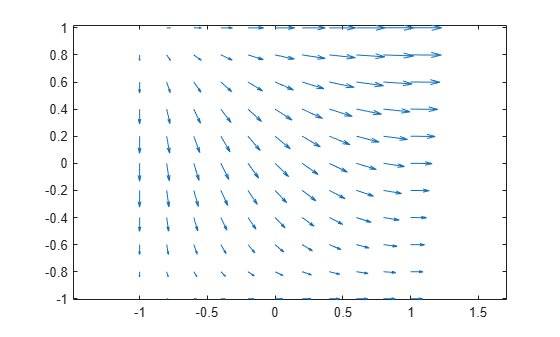Solve the heat transfer problem for the following 2-D geometry consisting of a square and a diamond made of different materials. Compute the heat flux, and plot it as a vector field.

Create a thermal model for transient analysis.

`thermalmodel = createpde('thermal','transient');`

Create the geometry and include it in the model.

```SQ1 = [3; 4; 0; 3; 3; 0; 0; 0; 3; 3]; D1 = [2; 4; 0.5; 1.5; 2.5; 1.5; 1.5; 0.5; 1.5; 2.5]; gd = [SQ1 D1]; sf = 'SQ1+D1'; ns = char('SQ1','D1'); ns = ns'; dl = decsg(gd,sf,ns); geometryFromEdges(thermalmodel,dl); pdegplot(thermalmodel,'EdgeLabels','on','FaceLabels','on') xlim([-1.5 4.5]) ylim([-0.5 3.5]) axis equal```For the square region, assign the following thermal properties: thermal conductivity is $10\phantom{\rule{0.16666666666666666em}{0ex}}W/\left({m}^{\circ }C\right)$, mass density is $2\phantom{\rule{0.16666666666666666em}{0ex}}kg/{m}^{3}$, and specific heat is $0.1\phantom{\rule{0.16666666666666666em}{0ex}}J/\left(k{g}^{\circ }C\right)$.

```thermalProperties(thermalmodel,'ThermalConductivity',10, ... 'MassDensity',2, ... 'SpecificHeat',0.1, ... 'Face',1);```

For the diamond-shaped region, assign the following thermal properties: thermal conductivity is $2\phantom{\rule{0.16666666666666666em}{0ex}}W/\left({m}^{\circ }C\right)$, mass density is $1\phantom{\rule{0.16666666666666666em}{0ex}}kg/{m}^{3}$, and specific heat is $0.1\phantom{\rule{0.16666666666666666em}{0ex}}J/\left(k{g}^{\circ }C\right)$.

```thermalProperties(thermalmodel,'ThermalConductivity',2, ... 'MassDensity',1, ... 'SpecificHeat',0.1, ... 'Face',2);```

Assume that the diamond-shaped region is a heat source with the density of $4\phantom{\rule{0.16666666666666666em}{0ex}}W/{m}^{3}$.

`internalHeatSource(thermalmodel,4,'Face',2);`

Apply a constant temperature of ${0}^{\circ }C$ to the sides of the square plate.

`thermalBC(thermalmodel,'Temperature',0,'Edge',[1 2 7 8]);`

Set the initial temperature to ${0}^{\circ }C$.

`thermalIC(thermalmodel,0);`

Mesh the geometry and solve the problem.

`generateMesh(thermalmodel);`

The dynamic for this problem is very fast: the temperature reaches steady state in about 0.1 seconds. To capture the interesting part of the dynamics, set the solution time to `logspace(-2,-1,10)`. This gives 10 logarithmically spaced solution times between 0.01 and 0.1. Solve the equation.

```tlist = logspace(-2,-1,10); thermalresults = solve(thermalmodel,tlist); temp = thermalresults.Temperature;```

Compute the heat flux density. Plot the solution with isothermal lines using a contour plot, and plot the heat flux vector field using arrows.

```[qTx,qTy] = evaluateHeatFlux(thermalresults); figure pdeplot(thermalmodel,'XYData',temp(:,10),'Contour','on', ... 'FlowData',[qTx(:,10) qTy(:,10)], ... 'ColorMap','hot')```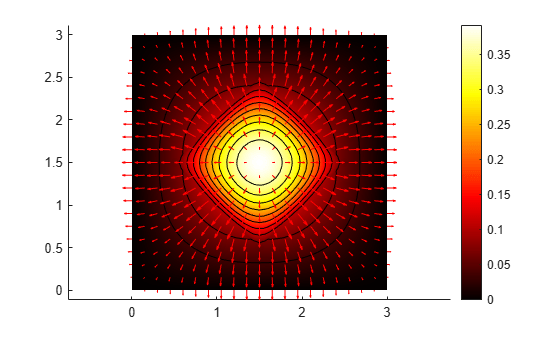## Input Arguments

collapse all

Solution of a thermal problem, specified as a `SteadyStateThermalResults` object or a `TransientThermalResults` object. Create `thermalresults` using the `solve` function.

Example: ```thermalresults = solve(thermalmodel)```

x-coordinate query points, specified as a real array. `evaluateHeatFlux` evaluates the heat flux at the 2-D coordinate points `[xq(i) yq(i)]` or at the 3-D coordinate points `[xq(i) yq(i) zq(i)]`. So `xq`, `yq`, and (if present) `zq` must have the same number of entries.

`evaluateHeatFlux` converts query points to column vectors `xq(:)`, `yq(:)`, and (if present) `zq(:)`. It returns the heat flux in a form of a column vector of the same size. To ensure that the dimensions of the returned solution are consistent with the dimensions of the original query points, use `reshape`. For example, use ```qx = reshape(qx,size(xq))```.

Data Types: `double`

y-coordinate query points, specified as a real array. `evaluateHeatFlux` evaluates the heat flux at the 2-D coordinate points `[xq(i) yq(i)]` or at the 3-D coordinate points `[xq(i) yq(i) zq(i)]`. So `xq`, `yq`, and (if present) `zq` must have the same number of entries.

`evaluateHeatFlux` converts query points to column vectors `xq(:)`, `yq(:)`, and (if present) `zq(:)`. It returns the heat flux in a form of a column vector of the same size. To ensure that the dimensions of the returned solution is consistent with the dimensions of the original query points, use `reshape`. For example, use ```qy = reshape(qy,size(yq))```.

Data Types: `double`

z-coordinate query points, specified as a real array. `evaluateHeatFlux` evaluates the heat flux at the 3-D coordinate points `[xq(i) yq(i) zq(i)]`. So `xq`, `yq`, and `zq` must have the same number of entries.

`evaluateHeatFlux` converts query points to column vectors `xq(:)`, `yq(:)`, and (if present) `zq(:)`. It returns the heat flux in a form of a column vector of the same size. To ensure that the dimensions of the returned solution is consistent with the dimensions of the original query points, use `reshape`. For example, use ```qz = reshape(qz,size(zq))```.

Data Types: `double`

Query points, specified as a real matrix with two rows for 2-D geometry or three rows for 3-D geometry. `evaluateHeatFlux` evaluates the heat flux at the coordinate points `querypoints(:,i)`, so each column of `querypoints` contains exactly one 2-D or 3-D query point.

Example: For 2-D geometry, ```querypoints = [0.5 0.5 0.75 0.75; 1 2 0 0.5]```

Data Types: `double`

Time indices, specified as a vector of positive integers. Each entry in `iT` specifies a time index.

Example: `iT = 1:5:21` specifies every fifth time-step up to 21.

Data Types: `double`

## Output Arguments

collapse all

x-component of the heat flux, returned as an array. The first array dimension represents the node index. The second array dimension represents the time step.

For query points that are outside the geometry, `qx` = `NaN`.

y-component of the heat flux, returned as an array. The first array dimension represents the node index. The second array dimension represents the time step.

For query points that are outside the geometry, `qy` = `NaN`.

z-component of the heat flux, returned as an array. The first array dimension represents the node index. The second array dimension represents the time step.

For query points that are outside the geometry, `qz` = `NaN`.

Introduced in R2017a

## SupportGet trial now